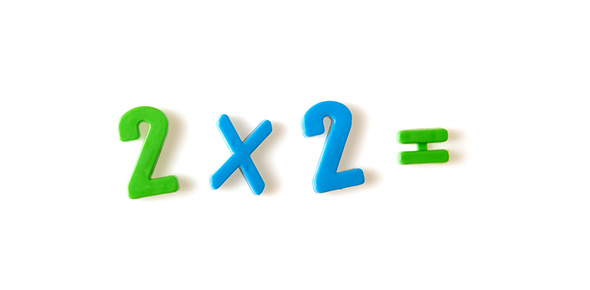# Morales 2.3 Multiplication Quiz

Approved & Edited by ProProfs Editorial Team
At ProProfs Quizzes, our dedicated in-house team of experts takes pride in their work. With a sharp eye for detail, they meticulously review each quiz. This ensures that every quiz, taken by over 100 million users, meets our standards of accuracy, clarity, and engagement.
| Written by Catherinehalcomb
C
Catherinehalcomb
Community Contributor
Quizzes Created: 1509 | Total Attempts: 5,237,716
Questions: 12 | Attempts: 239Settings• 1.

### 27 X 10 2

Explanation
The given multiplication problem is 27 multiplied by 10 to the power of 2. To solve this, we first calculate 10 to the power of 2, which is 100. Then, we multiply 27 by 100, resulting in 2,700.

Rate this question:

• 2.

### 80 X 102

Explanation
The given question is asking to multiply 80 by 102. The product of these two numbers is 8,160, not 8,000. Therefore, the given answer of 8,000 is incorrect.

Rate this question:

• 3.

### 306 X 102

Explanation
The given question is asking for the product of 306 and 102. To find the answer, we multiply 306 by 102, which equals 30,600. Therefore, the correct answer is 30,600.

Rate this question:

• 4.

### 145 X 102

Explanation
The given question asks for the product of 145 and 102. The correct answer is 14,500, which is obtained by multiplying 145 by 102.

Rate this question:

• 5.

### 520 X 102

Explanation
The given question asks for the product of 520 and 102. When multiplying these two numbers, we can multiply 520 by 2, which gives us 1040, and then multiply 520 by 100, which gives us 52,000. Therefore, the correct answer is 52,000.

Rate this question:

• 6.

### 60 X 103

Explanation
The given question is asking for the product of 60 multiplied by 103. The correct answer is 60,000. This is because when you multiply 60 by 103, you get a result of 6,180.

Rate this question:

• 7.

### 48 X 103

Explanation
The given question is asking for the product of 48 and 103. To find the answer, we multiply 48 by 103, which equals 4,944. Therefore, the correct answer is 4,944.

Rate this question:

• 8.

### 143 X 103

Explanation
The given question asks for the product of 143 and 103. To find the product, we multiply the two numbers together. When we multiply 143 by 103, we get 14,729. Therefore, the correct answer is 14,729.

Rate this question:

• 9.

### 630 X 103

Explanation
The given question asks for the product of 630 and 103. When multiplying these two numbers, we get 65,190. However, the correct answer provided is 630,000. It seems that there may be an error in the answer given, as it does not match the result of the multiplication.

Rate this question:

• 10.

### 35 X 105

Explanation
The correct answer is obtained by multiplying 35 by 105, which equals 3,675.

Rate this question:

• 11.

### 198 X 108

Explanation
The correct answer is obtained by multiplying 198 by 108. The product of these two numbers is 19,800, which can be represented as 19,800,000,000 in scientific notation.

Rate this question:

• 12.

### 256 X 102

Explanation
The given question is asking for the product of 256 and 102. To find the answer, we multiply 256 by 102, which gives us 25,600.

Rate this question:

Related TopicsBack to top
×

Wait!
Here's an interesting quiz for you.